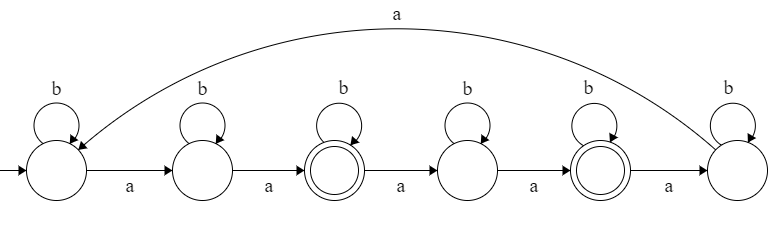# GATE | GATE CS 2020 | Question 61

Consider the following language.

`L = { x∈{a,b}* ∣ number of a’s in x divisible by 2 but not divisible by 3 } `

The minimum number of states in DFA that accepts L is _________ .

Note – This question was Numerical Type.
(A) 6
(B) 5
(C) 7
(D) 4

Explanation: Given language is,

```L = { x∈{a,b}* ∣ number of a’s in x divisible by 2 but not divisible by 3 }

L = L1 – L2 = L1 ∩ L2'

Where,
L1 = { x∈{a,b}* ∣ number of a’s in x divisible by 2 }
L2 = { x∈{a,b}* ∣ number of a’s in x divisible by 3 }

L2' = { x∈{a,b}* ∣ number of a’s not in x divisible by 3 } ```

Since L1 needed only 2 states and L2 needed only 3 states. Also, compliment of L2 (i.e., L2‘) needed same number of states as L2.

Therefore, L needed 2*3 = 6 states.Option (A) is correct.

Quiz of this Question

My Personal Notes arrow_drop_up
Article Tags :

Be the First to upvote.

Please write to us at contribute@geeksforgeeks.org to report any issue with the above content.